# KSEEB SSLC Class 10 Maths Solutions Chapter 3 Pair of Linear Equations in Two Variables Ex 3.2

KSEEB SSLC Class 10 Maths Solutions Chapter 3 Pair of Linear Equations in Two Variables Ex 3.2 are part of KSEEB SSLC Class 10 Maths Solutions. Here we have given Karnataka SSLC Class 10 Maths Solutions Chapter 3 Pair of Linear Equations in Two Variables Exercise 3.2.

## Karnataka SSLC Class 10 Maths Solutions Chapter 3 Pair of Linear Equations in Two Variables Exercise 3.2

Find the Value of x is used to consider unknown value.

Question 1.
Form the pair of linear equations in the following problems, and find their solutions graphically.
(i) 10 students of Class X took part in a Mathematics quiz. If the number of girls is 4 more than the number of boys, find the number of boys and girls who took part in the quiz.
(ii) 5 pencils and 7 pens together cost Rs. 50, whereas 7 pencils and 5 pens together cost Rs. 46. Find the cost of one pencil and that of one pen.
Solution:
(i) Strength of the X Std. is 10
Number of boys be ‘y’, then
number of girls be ‘x’ .
x + y = 10 …….. (i)
x = y + 4
∴ x – y = 4 ………. (ii)
From equations (i) + (ii)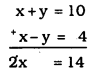$$\quad x=\frac{14}{2}=7$$
Substituting the value of ‘x’ in eqn. (i),
x + y = 10
7 + y = 10
y = 10 – 7
y = 4.
∴ Number of girls, x = 7
Number of boys, y = 4.

(ii) Cost of each pencil be Rs. ‘x’
Cost of pen be Rs. ‘y’
5x + 7y = 50 ………. (i)
7x + 5y = 46 ……….. (ii)
From equation (i) + equation (ii)∴ x + y = 8 …………… (iii)
from Eqn. (ii) – Eqn. (i),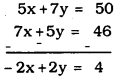∴ -x + y = 2 …………. (iv)
Eqn. (iii) + Eqn. (iv)∴ y = 5
Substituting the value of ‘y’ in eqn. (i)
x + y = 8
x + 5 = 8
∴ x = 8 – 5 x = 3
∴Cost of each pencil is Rs. 3.
Cost of each pen is Rs. 5.

Question 2.
On comparing the ratios $$\frac{a_{1}}{a_{2}}, \frac{b_{1}}{b_{2}} \text { and } \frac{c_{1}}{c_{2}}$$ find out whether the lines representing the following pairs of linear equations intersect at a point, are parallel or coincident:
i) 5x – 4y + 8 = 0
7x + 6y – 9 = 0
ii) 9x + 3y + 12 = 0
18x + 6y + 24 = 0
iii) 6x – 3y + 10 = 0
2x – y + 9 = 0
Solution:
i) 5x – 4y + 8 = 0 a1= 5, b1 = -4, C1= 8
7x + 6y – 9 = 0 a2 = 7, b2= 6, c2= -9
$$\frac{a_{1}}{a_{2}}=\frac{5}{7} \quad \frac{b_{1}}{b_{2}}=\frac{-4}{6} \quad \frac{c_{1}}{c_{2}}=\frac{8}{-9}$$
Here, $$\frac{\mathbf{a}_{1}}{\mathbf{a}_{2}} \neq \frac{\mathbf{b}_{1}}{\mathbf{b}_{2}}$$
∴ Lines representing the pairs of linear equations intersect at a point.

ii) 9x + 3y + 12 = 0
18x + 6y + 24 = 0
a1 =9, b1 = 3, c1 = 12
a2 = 18, b2 = 6, c2 = 24
$$\frac{\mathrm{a}_{1}}{\mathrm{a}_{2}}=\frac{9}{18}=\frac{1}{2} \quad \frac{\mathrm{b}_{1}}{\mathrm{b}_{2}}=\frac{3}{6}=\frac{1}{2}$$
$$\frac{c_{1}}{c_{2}}=\frac{12}{24}=\frac{1}{2}$$
Here, $$\frac{\mathrm{a}_{1}}{\mathrm{a}_{2}}=\frac{\mathrm{b}_{1}}{\mathrm{b}_{2}}=\frac{\mathrm{c}_{1}}{\mathrm{c}_{2}}$$
∴ Representation of lines graphically are coincident.

iii) 6x – 3y + 10 = 0
2x – y + 9 = 0
Here, a1 = 6, b1 = -3, c1 = 10
a2 = 2, b2 = -1, c2 = 9
$$\frac{a_{1}}{a_{2}}=\frac{6}{2}=\frac{3}{1} \quad \frac{b_{1}}{b_{2}}=\frac{-3}{-1}=\frac{3}{1}$$
$$\frac{c_{1}}{c_{2}}=\frac{10}{9}$$
Here, $$\frac{a_{1}}{a_{2}}=\frac{b_{1}}{b_{2}} \neq \frac{c_{1}}{c_{2}}$$
∴ Representation of lines graphically is parallel.

Question 3.
On compairing the ratios $$\frac{\mathrm{a}_{1}}{\mathrm{a}_{2}}, \frac{\mathrm{b}_{1}}{\mathrm{b}_{2}}$$ and $$\frac{c_{1}}{c_{2}}$$ find out whether the following pair of linear equations are consistent, or inconsistent.
i) 3x + 2y = 5; 2x – 3y = 7
ii) 2x – 3y = 8; 4x-6y = 9
iii) $$\frac{3}{2} x+\frac{5}{3} y=7 : 9 x-10 y=14$$
iv) 5x – 3y = 11: -10x – 6y = -22
v) $$\frac{4}{3} x+2 y=8 ; 2 x+3 y=12$$
Solution:
i) 3x + 2y = 5 ⇒ 3x + 2y – 5 = 0
2x – 3y = 7 ⇒ 2x – 3y – 7 = 0
Here, a1 = 3, b1 = 2, c1 = -5
a2 = 2, b2 = -3, c2 = -7
$$\frac{a_{1}}{a_{2}}=\frac{3}{2} \quad \frac{b_{1}}{b_{2}}=-\frac{2}{3} \quad \frac{c_{1}}{c_{2}}=\frac{-5}{-7}=\frac{5}{7}$$
Here, $$\frac{a_{1}}{a_{2}} \neq \frac{b_{1}}{b_{2}}$$
∴ Graphical representation is intersecting lines.
∴ Pair of linear equations are consistent.

ii) 2x – 3y = 8 ⇒ 2x – 3y – 8 = 0
4x – 6y = 9 ⇒ 4x – 6y – 9 = 0
a1 = 2, b1 = -3, c1 = -8
a2 = 4, b2 = -6, c2 = -9
$$\frac{a_{1}}{a_{2}}=\frac{2}{4}=\frac{1}{2} \quad \frac{b_{1}}{b_{2}}=\frac{-3}{-6}=\frac{1}{2}$$
$$\frac{c_{1}}{c_{2}}=\frac{-8}{-9}=\frac{8}{9}$$
Here, $$\frac{\mathrm{a}_{1}}{\mathrm{a}_{2}}=\frac{\mathrm{b}_{1}}{\mathrm{b}_{2}} \neq \frac{\mathrm{c}_{1}}{\mathrm{c}_{2}}$$
∴ Graphical representation is parallel lines.
∴ Equations are inconsistent.

iii) $$\frac{3}{2} x+\frac{5}{3} y=7 \quad \frac{3}{2} x+\frac{5}{3} y-7=0$$
9x – 10y = 14 ⇒ 9x – 10y – 14 = 0
$$a_{1}=\frac{3}{2}, \quad b_{1}=\frac{5}{3}, \quad c_{1}=-7$$
a2 = 9, b2 = -10, c2 = -17
$$\frac{a_{1}}{a_{2}}=\frac{3}{2} \times \frac{1}{9} \quad \frac{b_{1}}{b_{2}}=\frac{5}{3} \times \frac{-1}{6}$$
$$\frac{c_{1}}{c_{2}}=\frac{-7}{-14}=\frac{7}{14}=\frac{1}{2}$$
Here, $$\frac{a_{1}}{a_{2}} \neq \frac{b_{1}}{b_{2}}$$
∴ Pair of equations are consistent

iv) 5x – 3y = 11 ⇒ 5x – 3y – 11 = 0
-10x + 6y = – 22 ⇒ -10x + 6y + 22 = 0
a1 = 5, b1 = -3, c1 = -11
a2 = -10, b2 = 6, c2 = -22
$$\frac{a_{1}}{a_{2}}=\frac{5}{-10}=-\frac{1}{2} \quad \frac{b_{1}}{b_{2}}=\frac{-3}{6}=-\frac{1}{2}$$
$$\frac{c_{1}}{c_{2}}=\frac{-11}{22}=-\frac{1}{2}$$
Here, $$\frac{a_{1}}{a_{2}}=\frac{b_{1}}{b_{2}}=\frac{c_{1}}{c_{2}}$$
∴ Pair of equations are consistent
∴ Graphical representation is coninciding.

v) $$\frac{4}{3} x+2 y=8 \quad \frac{4}{3} x+26-8=0$$
2x + 3y = 12 ⇒ 2x + 3y – 12 = 0
$$a_{1}=\frac{4}{3}, \quad b_{1}=2, \quad c_{1}=-8$$
a2 = 2, b2 = 3, c2 = -12
$$\frac{a_{1}}{a_{2}}=\frac{4}{3} \times \frac{1}{2}=\frac{1}{6} \quad \frac{b_{1}}{b_{2}}=\frac{2}{3}$$
$$\frac{c_{1}}{c_{2}}=\frac{-8}{-12}=\frac{2}{3}$$
Here, $$\frac{\mathbf{a}_{1}}{\mathbf{a}_{2}} \neq \frac{\mathbf{b}_{1}}{\mathbf{b}_{2}}$$
∴ Pair of equations are consistent

Question 4.
Which of the following pairs of linear equations are consistent/inconsistent? If consistent obtain the solution graphically
(i) x + y = 5, 2x + 2y = 10
(ii) x-y = 8 3x-3y= 16
(iii) 2x + y – 6 = 0 4x – 2y – 4 = 0
(iv) 2x – 2y – 2 = 0 4x – 3y – 5 = 0
Solution:
(i) x + y = 5 ⇒ x + y – 5 = 0
2x + 2y = 10 ⇒ 2x + 2y – 10 = 0
a1 = 1, b1 = 1, c1 = -5
a2 = 2, b2 = 2, c2 = -10
$$\frac{\mathrm{a}_{1}}{\mathrm{a}_{2}}=\frac{1}{2} \quad \frac{\mathrm{b}_{1}}{\mathrm{b}_{2}}=\frac{1}{2} \quad \frac{\mathrm{c}_{1}}{\mathrm{c}_{2}}=\frac{-5}{-10}=\frac{1}{2}$$
Here, $$\frac{\mathrm{a}_{1}}{\mathrm{a}_{2}}=\frac{\mathrm{b}_{1}}{\mathrm{b}_{2}}=\frac{\mathrm{c}_{1}}{\mathrm{c}_{2}}$$
∴ Pair of equations are consistent
(i) x + y = 5
y = 5 – x

 x 0 2 4 y = 5 – x 5 3 1

(ii) 2x + 2y = 10
x + y = 5
y = 5 – x

 x 0 2 5 y = 5 – x 5 3 0

∴ We can give any value for ‘x’, i.e., solutions are infinite.
∴ Solution, P (5, 0) x = 5, y = 0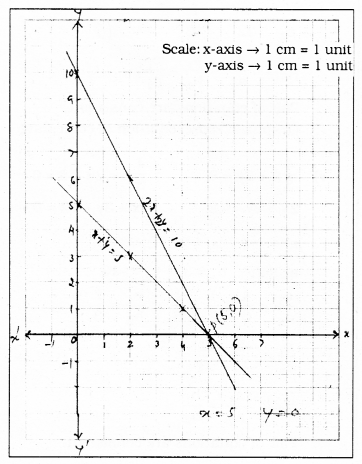(ii) x – y = 8 ⇒ x – y – 8 = 0
3x – 3y = 16 ⇒ 3x – 3y – 16 = 0
Here, $$\frac{a_{1}}{a_{2}}=\frac{1}{3} \quad \frac{b_{1}}{b_{2}}=\frac{-1}{-3}=\frac{1}{3}$$
$$\frac{c_{1}}{c_{2}}=\frac{-8}{-16}=\frac{1}{2}$$
$$\quad \frac{\mathrm{a}_{1}}{\mathrm{a}_{2}}=\frac{\mathrm{b}_{1}}{\mathrm{b}_{2}} \neq \frac{\mathrm{c}_{1}}{\mathrm{c}_{2}}$$
∴ Linear equations are inconsistenttent.
∴ Algebraically it has no solution.
Graphical representation → Parallel Lines.
(i) x – y = 8
-y = 8 – x
y = -8 + x

 x 8 10 9 y = -8+x 0 2 1

(ii) 3x – 3y = 16
-3y = 16 – 3x
3y = -16 + 3x
$$\quad y=\frac{-16+3 x}{3}$$

 x 6 8 $$y=\frac{-16+3 x}{3}$$ 0.8 2.6

No solution because it is inconsistent(iii) 2x + y – 6 = 0
4x – 2y – 4 = 0
Here a1 = 2, b1 = 1, c1 = -6
a2 = 4, b2 = -2, c2 = -4
$$\frac{\mathrm{a}_{1}}{\mathrm{a}_{2}}=\frac{2}{4}=\frac{1}{2} \quad \frac{\mathrm{b}_{1}}{\mathrm{b}_{2}}=\frac{1}{-2}$$
$$\frac{c_{1}}{c_{2}}=\frac{-6}{-4}=\frac{3}{2}$$
Here, $$\frac{a_{1}}{a_{2}} \neq \frac{b_{1}}{b_{2}}$$
Pair of equations are consistent. Algebraically both lines intersect.
Graphical Representation :
(i) 2x + y = 6
y = 6 – 2x

 x 0 2 y = 6 – 2x 6 2

(ii) 4x – 2y – 4 = 0
4x – 2y = 4
-2y = 4 – 4x
2y = -4 + 4x
$$\quad y=\frac{-4+4 x}{2}$$

 x 1 3 $$y=\frac{-4+4 x}{2}$$ 0 4

Solution: intersecting point, P (2, 2) i.e., x = 2, y = 2(iv) 2x – 2y – 2 = 0
4x – 3y – 5 = 0
a1 = 2, b1 = -2, c1 = -2
a2 = 4, b2 = -3, c2 = -5
$$\frac{\mathrm{a}_{1}}{\mathrm{a}_{2}}=\frac{2}{4}=\frac{1}{2} \quad \frac{\mathrm{b}_{1}}{\mathrm{b}_{2}}=\frac{-2}{-3}=\frac{2}{3}$$
$$\frac{c_{1}}{c_{2}}=\frac{-2}{-5}=\frac{2}{5}$$
Here, $$\frac{\mathrm{a}_{1}}{\mathrm{a}_{2}} \neq \frac{\mathrm{b}_{1}}{\mathrm{b}_{2}}$$
Pair of equations are consistent.
∴ Algebraically both lines intersect.
Graphical Representation :
(i) 2x – 2y – 2 =0
2x – 2y = 2
-2y = 2 – 2x
2y = -2 + 2x
$$\quad y=\frac{-2+2 x}{2}$$
∴ y = – 1 + x

 x 2 4 y= -1+ x 1 3

(ii) 4x – 3y – 5 = 0
4x – 3y = 5
-3y = 5 – 4x
3y = -5 + 4x
$$\quad y=\frac{-5+4 x}{3}$$

 x 2 5 $$y=\frac{-5+4 x}{3}$$ 1 5

Solution: P(2, 1) i.e., x = 2, y = 1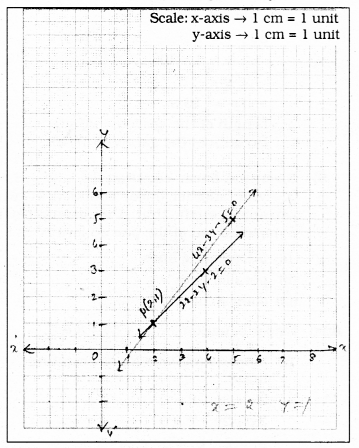Question 5.
Half the perimeter of a rectangular garden, whose length is 4m more than its width, is 36m. Find the dimensions of the garden.Solution:
Length of rectangular garden be ‘x’ m.
Breadth of rectangular garden be ‘y’ m, then
Length of the garden is 4m more than its width.
x = y + 4 ……….. (i)
x – y = 4
Half of the circumference is 36 m.
$$\frac{2 x+2 y}{2}=36$$
2x + 2y = 72
x + y = 36 …………… (ii)
∴ x – y = 4 (i)
x + y = 36 (ii)
From eqn. (i) + eqn. (ii)$$\quad x=\frac{40}{2}$$
∴ x = 20 m.
Substituting the value of ’x’ in eqn. (i)
x – y = 4
20 – y = 4
-y = 4 – 20
-y = -16
y = 16 m.
∴ Length of the garden = 20 m.
Breadth of the garden = 16 m.

Question 6.
Given the linear equation 2x + 3y – 8 = 0, write another linear equation in two variables such that the geometrical representation of the pair so formed is :
(i) intersecting lines
(ii) parallel lines
(iii) coincident lines.
Solution:
Linear equation is 2x + 3y – 8 = 0.
(i) Linear equations for intersecting lines:
2x + 3y – 8 = 0
3x + 2y – 7 = 0
a1 = 2, b1 = 3, c1 = -8
a2 = 3, b2 = 2, c2 = -7
$$\frac{a_{1}}{a_{2}}=\frac{2}{3} \quad \frac{b_{1}}{b_{2}}=\frac{3}{2}$$
Here, when $$\frac{\mathrm{a}_{1}}{\mathrm{a}_{2}} \neq \frac{\mathrm{b}_{1}}{\mathrm{b}_{2}}$$ Geometrical representation is intersecting lines.

(ii) For Parallel lines :
2x + 3y – 8 = 0
2x + 3y – 12 = 0
a1 = 2, b1 = 3, c1= -8
a1 = 2, b1 = 3, c1 = -12
$$\frac{\mathrm{a}_{1}}{\mathrm{a}_{2}}=\frac{2}{2}=\frac{1}{1} \quad \frac{\mathrm{b}_{1}}{\mathrm{b}_{2}}=\frac{3}{3}=\frac{1}{1}$$
$$\frac{c_{1}}{c_{2}}=\frac{-8}{-12}=\frac{8}{12}=\frac{2}{3}$$
Here, $$\frac{\mathrm{a}_{1}}{\mathrm{a}_{2}}=\frac{\mathrm{b}_{1}}{\mathrm{b}_{2}} \neq \frac{\mathrm{c}_{1}}{\mathrm{c}_{2}}$$ hence
Graphical representation is parallel lines.

(iii) For Intersecting lines :
2x + 3y – 8 = 0
4x + 6y – 16 = 0
a1 = 2, b1 = 3, c1 = -8
a1 = 4, b1 = 6, c1 = -16
$$\frac{a_{1}}{a_{2}}=\frac{2}{4}=\frac{1}{2} \quad \frac{b_{1}}{b_{2}}=\frac{3}{6}=\frac{1}{2}$$
$$\frac{c_{1}}{c_{2}}=\frac{-8}{-16}=\frac{1}{2}$$
Here,
∴ Lines are intersecting.

Question 7.
Draw the graphs of the equations x – y + 1 =0 and 3x + 2y – 12 = 0. Determine the coordinates of the vertices of the triangle formed by these lines and the x-axis, and shade the triangular region.
Solution:
x – y + 1 = 0 ………. (i)
3x – 2y – 12 = 0 ……….. (ii)
x – y + 1 = 0
-y = -x -1
y = x + 1

 x 2 4 y = x + 1 3 5

3x + 2y – 12 = 0
2y = -3x + 12
$$\quad y=\frac{-3 x+12}{2}$$

 x 0 2 $$y=\frac{-3 x+12}{2}$$ 6 3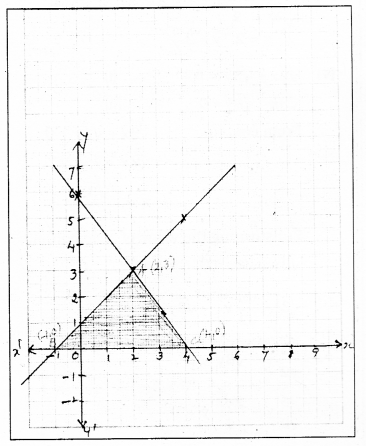Graphs of these two equations intersect at A. Vertices formed for ∆ABC are,
A (2, 3), B (-1, 0), C (4, 0).

We hope the given KSEEB SSLC Class 10 Maths Solutions Chapter 3 Pair of Linear Equations in Two Variables Ex 3.2 will help you. If you have any query regarding Karnataka SSLC Class 10 Maths Solutions Chapter 3 Pair of Linear Equations in Two Variables Exercise 3.2, drop a comment below and we will get back to you at the earliest.

error: Content is protected !!